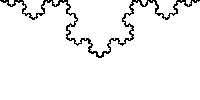### Fractal Speed

What is the maximum speed a fractal can be produced?
The fractal is produced at the fractal processing speed (fractal speed), this is the speed at which a discernible fractal shape can be created. This speed also determines the speed of zoom – or magnification into the fractal. It is also the speed of the fractal – wave.Fractal zoom

Fractal speed can be demonstrated by drawing the Koch snowflake freehand. This is rather slow and timely; a much faster method is with modern computer as shown below. The speed is thus limited by the processing power of production. I have published in early entries on the production of the fractal. The average modern computer (in 2011) cannot produce many more than 7 iterations  –  in one  view or 'fractal paradigm'  – before the computer crashes. To produce more, or see more, we must zoom – forward and into and fractal.
The maximum zoom fractal speed must be 'Maxwell’s'  – speed of light.
If this is so, then special relativity should also be consistent with the fractal as the fractal demonstrates increasing cost with the more iterations or production. The extra – marginal –  cost, is mass in relativity. See diagram below:  mass or cost limits the production speed.
It maybe that at the maximum fractal speed – the speed of light? –  the fractal is also at a 'perfect' state of superposition where there is no reference points –  and thus no connection to time.